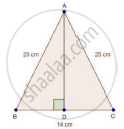Share

# In an Isosceles Triangle Abc, Ab = Ac = 25 Cm, Bc = 14 Cm. Calculate the Altitude from a on Bc. - CBSE Class 10 - Mathematics

ConceptApplication of Pythagoras Theorem in Acute Angle and Obtuse Angle

#### Question

In an isosceles triangle ABC, AB = AC = 25 cm, BC = 14 cm. Calculate the altitude from A on BC.

#### SolutionWe have

AB = AC = 25 cm and BC = 14 cm

In ΔABD and ΔACD

AB = AC [Each 25 cm]

Then, ΔABD ≅ ΔACD [By RHS condition]

∴ BD = CD = 7 cm [By c.p.c.t]

⇒ AD2 + 72 = 252

⇒ AD2 = 625 − 49 = 576

⇒ AD = sqrt576 = 24 cm

Is there an error in this question or solution?

#### Video TutorialsVIEW ALL 

Solution In an Isosceles Triangle Abc, Ab = Ac = 25 Cm, Bc = 14 Cm. Calculate the Altitude from a on Bc. Concept: Application of Pythagoras Theorem in Acute Angle and Obtuse Angle.
S